Hybrid stochastic kinetic description of two-dimensional traffic dynamics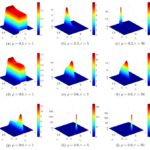Michael Herty, Andrea Tosin, Giuseppe Visconti, Mattia Zanella

SIAM Journal on Applied Mathematics, 78(5): 2737–2762, 2018. (Preprint arXiv)

In this work we present a two-dimensional kinetic traffic model which takes into account speed changes both when vehicles interact along the road lanes and when they change lane. Assuming that lane changes are less frequent than interactions along the same lane and considering that their mathematical description can be done up to some uncertainty in the model parameters, we derive a hybrid stochastic Fokker-Planck-Boltzmann equation in the quasi-invariant interaction limit. By means of suitable numerical methods, precisely structure preserving and direct Monte Carlo schemes, we use this equation to compute theoretical speed-density diagrams of traffic both along and across the lanes, including estimates of the data dispersion, and validate them against real data.

Control strategies for road risk mitigation in kinetic traffic modelling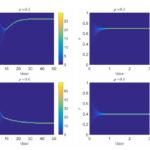Andrea Tosin, Mattia Zanella.

IFAC-PapersOnLine, 51(9): 67-72, 2018. (Preprint arXiv)

In this paper we present a Boltzmann-type kinetic approach to the modelling of road traffic, which includes control strategies at the level of microscopic binary interactions aimed at the mitigation of speed-dependent road risk factors. Such a description is meant to mimic a system of driver-assist vehicles, which by responding locally to the actions of their drivers can impact on the large-scale traffic dynamics, including those related to the collective road risk and safety.

Boltzmann-type models with uncertain binary interactions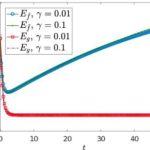Andrea Tosin, Mattia Zanella.

Communications in Mathematical Sciences, 16(4): 963-985, 2018. (Preprint arXiv)

In this paper we study binary interaction schemes with uncertain parameters for a general class of Boltzmann-type equations with applications in classical gas and aggregation dynamics. We consider deterministic (i.e., a priori averaged) and stochastic kinetic models, corresponding to different ways of understanding the role of uncertainty in the system dynamics, and compare some thermodynamic quantities of interest, such as the mean and the energy, which characterise the asymptotic trends. Furthermore, via suitable scaling techniques we derive the corresponding deterministic and stochastic Fokker-Planck equations in order to gain more detailed insights into the respective asymptotic distributions. We also provide numerical evidences of the trends estimated theoretically by resorting to recently introduced structure preserving uncertainty quantification methods.

Uncertainty quantification for kinetic models in socio-economic and life sciences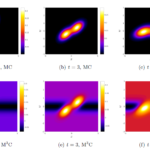Giacomo Dimarco, Lorenzo Pareschi, Mattia Zanella

Uncertainty Quantification for Hyperbolic and Kinetic Equations, Eds. S. Jin, L. Pareschi, SEMA-SIMAI Springer Series vol.14, Springer-Cham, 2017. (Preprint arXiv)

Kinetic equations play a major rule in modeling large systems of interacting particles. Recently the legacy of classical kinetic theory found novel applications in socio-economic and life sciences, where processes characterized by large groups of agents exhibit spontaneous emergence of social structures. Well-known examples are the formation of clusters in opinion dynamics, the appearance of inequalities in wealth distributions, flocking and milling behaviors in swarming models, synchronization phenomena in biological systems and lane formation in pedestrian traffic. The construction of kinetic models describing the above processes, however, has to face the difficulty of the lack of fundamental principles since physical forces are replaced by empirical social forces. These empirical forces are typically constructed with the aim to reproduce qualitatively the observed system behaviors, like the emergence of social structures, and are at best known in terms of statistical information of the modeling parameters. For this reason the presence of random inputs characterizing the parameters uncertainty should be considered as an essential feature in the modeling process. In this survey we introduce several examples of such kinetic models, that are mathematically described by nonlinear Vlasov and Fokker–Planck equations, and present different numerical approaches for uncertainty quantification which preserve the main features of the kinetic solution.

Structure preserving schemes for nonlinear Fokker-Planck equations and applications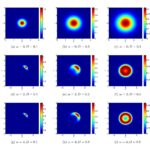Lorenzo Pareschi, Mattia Zanella

Journal of Scientific Computing, 74(3): 1575-1600, 2018. (Preprint arXiv)

In this paper we focus on the construction of numerical schemes for nonlinear Fokker-Planck equations that preserve the structural properties, like non negativity of the solution, entropy dissipation and large time behavior. The methods here developed are second order accurate, they do not require any restriction on the mesh size and are capable to capture the asymptotic steady states with arbitrary accuracy. These properties are essential for a correct description of the underlying physical problem. Applications of the schemes to several nonlinear Fokker-Planck equations with nonlocal terms describing emerging collective behavior in socio-economic and life sciences are presented

Recent advances in opinion modeling: control and social influence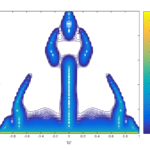Giacomo Albi, Lorenzo Pareschi, Giuseppe Toscani, Mattia Zanella

Active Particles, Volulme 1. Advances in Theory, Models, and Applications, pp. 49-98, 2017. (Preprint arXiv)

We survey some recent developments on the mathematical modeling of opinion dynamics. After an introduction on opinion modeling through interacting multi-agent systems described by partial differential equations of kinetic type, we focus our attention on two major advancements: optimal control of opinion formation and influence of additional social aspects, like conviction and number of connections in social networks, which modify the agents’ role in the opinion exchange process.

Kinetic models of collective decision-making in the presence of equality bias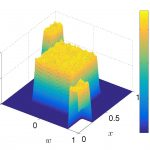Lorenzo Pareschi, Pierluigi Vellucci, Mattia Zanella

Physica A: Statistical Mechanics and its Applications, 10(1):1-32, 2017. (Preprint arXiv)

We introduce and discuss kinetic models describing the influence of the competence in the evolution of decisions in a multi-agent system. The original exchange mechanism, which is based on the human tendency to compromise and change opinion through self-thinking, is here modified to include the role of the agents’ competence. In particular, we take into account the agents’ tendency to behave in the same way as if they were as good, or as bad, as their partner: the so-called equality bias. This occurred in a situation where a wide gap separated the competence of group members. We discuss the main properties of the kinetic models and numerically investigate some examples of collective decision under the influence of the equality bias. The results confirm that the equality bias leads the group to suboptimal decisions.

Opinion dynamics over complex networks: kinetic modelling and numerical methods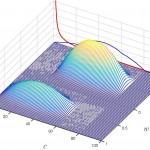Giacomo Albi, Lorenzo Pareschi, Mattia Zanella

Kinetic and Related Models, 10(1): 1-32, 2017. (Preprint arXiv)

In this paper we consider the modeling of opinion dynamics over time dependent large scale networks. A kinetic description of the agents’ distribution over the evolving network is considered which combines an opinion update based on binary interactions between agents with a dynamic creation and removal process of new connections. The number of connections of each agent influences the spreading of opinions in the network but also the way connections are created is influenced by the agents’ opinion. The evolution of the network of connections is studied by showing that its asymptotic behavior is consistent both with Poisson distributions and truncated power-laws. In order to study the large time behavior of the opinion dynamics a mean field description is derived which allows to compute exact stationary solutions in some simplified situations. Numerical methods which are capable to describe correctly the large time behavior of the system are also introduced and discussed. Finally, several numerical examples showing the influence of the agents’ number of connections in the opinion dynamics are reported.

Performance bounds for the mean-field limit of constrained dynamics

Discrete and Continuous Dynamical Systems – Series A, 37(4): 2023-2043, 2017. (Preprint arXiv)

In this work we are interested in the mean-field formulation of kinetic models under control actions where the control is formulated through a model predictive control strategy (MPC) with varying horizon. The relation between the (usually hard to compute) optimal control and the MPC approach is investigated theoretically in the mean-field limit. We establish a computable and provable bound on the difference in the cost functional for MPC controlled and optimal controlled system dynamics in the mean-field limit. The result of the present work extends previous findings for systems of ordinary differential equations. Numerical results in the mean-field setting are given.

On the optimal control of opinion dynamics on evolving networks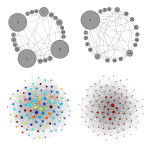Giacomo Albi, Lorenzo Pareschi, Mattia Zanella

In: Bociu L., Désidéri JA., Habbal A. (eds) System Modeling and Optimization. CSMO 2015. IFIP Advances in Information and Communication Technology, vol 494. Springer, Cham. (Preprint arXiv)

In this work we are interested in the modelling and control of opinion dynamics spreading on a time evolving network with scale-free asymptotic degree distribution. The mathematical model is formulated as a coupling of an opinion alignment system with a probabilistic description of the network. The optimal control problem aims at forcing consensus over the network, to this goal a control strategy based on the degree of connection of each agent has been designed. A numerical method based on a model predictive strategy is then developed and different numerical tests are reported. The results show that in this way it is possible to drive the overall opinion toward a desired state even if we control only a suitable fraction of the nodes.